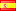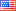# What is new in PHP 5.6?PHP is not a language that has been forgotten or abandoned, on the contrary it is in constant evolution and in this way its development team shown us with the latest release due PHP-5.6 adds new features that make more easy the development process. Within these new features, we can mention:

• Constant expressions
• Variadic functions / New operator
• New exponentiation operator **
• Argument unpacking via
• use function and use const
• Interactive debugger: phpdbg
• Other

## Constant expressions

Now the constants support expressions involving scalars, numeric, strings, constants and arrangements.

Definition of constant that uses another constant with numeric operations

``````<?php
const UNO = 1;
const DOS = UNO * 2; // This was not possible before versión 5.6
// Print 2;
echo DOS, '<br/>';
``````

Definition of constant inside a class involving a global constant, friend constant (constants defined in the same class), scalar and string operations.

``````<?php
class C {
const TRES = DOS + 1;
const UN_TERCIO = UNO / self::TRES;
const LIBRE = 'Libre';
const LIBRE_BYTE = self::LIBRE . 'Byte';
}

// It prints 3;
echo C::TRES,  '<br/>';
// Print 0.3333...
echo C::UN_TERCIO, '<br/>';
// Print LibreByte
echo C::LIBRE_BYTE, '<br/>';
``````

It is now possible to define arrays of constants which, in turn, involve other constants and other array of constants

``````<?php
/* Array of constants */
const ARR = ['A', 'B', 'C'];
/* Multiple constants array */
const MULTI_ARR = [[1,2], ['A', 'B'], 'C'];
/* Array of constants involving another array of constant and a constant inside a class */
const MIX_ARR = [ARR, C::LIBRE_BYTE];

// Print B
echo ARR, '<br/>';
// Print 2
echo MULTI_ARR;
// Print LibreByte
echo MIX_ARR;
``````

The variadic functions are functions that support a variable number of arguments. Before PHP-5.6 this was achieved by using func_num_args (), func_get_arg(), and func_get_args() functions, with the release of PHP-5.6 you can use the operator to indicate that the function supports a variable number of arguments.

For example if we would like to calculate the average of a baseball player we can do it with the following function

``````<?php
function avg(...\$veces_al_bate) {
return (array_sum(\$veces_al_bate) / count(\$veces_al_bate)) * 1000;
}

// Print 300
echo avg(1, 0, 1, 0, 0, 0, 0, 1, 0, 0);
``````

Before PHP-5.6 would do it in the following way:

``````<?php
function avg() {
return (array_sum(func_get_args()) / func_num_args()) * 1000;
}
// Print 300
echo avg(1, 0, 1, 0, 0, 0, 0, 1, 0, 0);
``````

It is also possible to specify arguments before the operator In this case, only the arguments that do not match the specified arguments will be added to the array generated by the operator If before the operator you specify a “hint” then all the arguments captured by they must be of the specified type else “fatal error” is generated. For example we want to implement a function that allows you to format one or several dates at the same time then would have something like:

``````<?php
function fmt_fechas(\$format, DateTime ...\$dates) {
// array_map recorre los elementos del arreglo y le aplica la
return array_map(function (\$date) use (\$format) {
return \$date->format(\$format);
}, \$dates);
}
// Imprime:
// { => string(10) "2015-07-03" => string(10) "2015-07-03" => string(10) "2015-07-03" }
var_dump(fmt_fechas('Y-m-d', new DateTime(), new DateTime('-3 days'),  new DateTime('+1 month')));
// Imprime:
// Catchable fatal error: Argument 4 passed to fmt_fechas() must be an instance of DateTime, null given...
fmt_fechas('Y-m-d', new DateTime(), new DateTime('-3 days'), null);
``````

## Exponentiation via **

Now it is possible to calculate the power of a number using the operator **, for example to calculate 2 power 8, you would do the following:

``````<?php
// Print 256
echo 2 ** 8;
``````

**= is the shorthand assignment operator for ** operator

``````<?php
\$a = 2 ** 1;
\$a **= 8;
// Print 256
echo \$a, '<br/>';
``````

## Argument unpacking via …

It is also possible to use the operator to convert an array or variable Traversable or literal in a list of arguments. This new functionality particularly I like much because before PHP-5.6 if we had an array of values, and we wanted to pass them as parameters first we unreference the array.

Before PHP-5.6:

``````<?php
function sum(\$a, \$b) {
return \$a + \$b;
}

\$a = [1, 2];

// We desreferencing the  array
// Print 3
echo sum(\$a, \$a), '<br/>';
``````

From PHP-5.6 would be sufficient:

``````<?php
function sum(\$a, \$b) {
return \$a + \$b;
}

// Print 3
echo sum(...[1, 2]), '<br />';

\$a = [1, 2];

// Print 3
echo sum(...\$a), '<br />';
``````

## use function and use const

From PHP-5.6, it is possible to import constants and functions specific to a name space by making use of the constructions: use const, use function, if we have the following namespace definition.

``````<?php
namespace Mi\Espacio\DeNombre
{
const MI_CONSTANTE = 'MI_CONSTANTE';
function miFuncion()
{
echo __FUNCTION__, '<br/>';
}
}
``````

We can import MI_CONSTANTE and miFuncion with the following definition:

``````<?php
namespace Cliente {
use const MiEspacio\DeNombre\MI_CONSTANTE;
use function MiEspacio\DeNombre\miFuncion;

// Print: MI_CONSTANTE
echo MI_CONSTANTE, '<br/>';
// Print: MiEspacio\DeNombre\miFuncion;
miFuncion();
}
``````

## Interactive debugger: phpdbg

PHP-5.6 includes an interactive debugger called phpdbg implemented as a SAPI module, phpdbg allows total control of the environment upon which executes without impact on the functionality and performance on debugged code. For more information, see the documentation for phpdbg.

## Default encoding

Now the htmlentities(), html_entity_decode(), htmlspecialchars() use by default the value assigned to the directive: default_charset

Files larger than 2 gigabytes in size are now accepted.

To know all news about PHP-5.6 see: New features

## Obsolete features

Call from incompatible context. The methods called from incompatible context now are obsolete, generating E_DEPRECATED instead of E_STRICT errors. Calling methods from incompatible contexts will be removed in future versions of PHP.

``````<?php
class A
{
function funcionA()
{
echo A::class;
}
}

class B
{
function funcionB()
{
A::funcionA();
}
}

(new B)->funcionB();
``````

Note that funcionA is being called statically (and has not been declared as static) from an instance of the class B then the contexts are not compatible and E_DEPRECATED error is generated

``````Deprecated: Non-static method A::funcionA() should not be called statically,
assuming \$this from incompatible context in ...
``````

If we called in this way:

``````<?php
B::funcionB();
``````

Then an E_STRICT error message would be generated

``````Strict Standards: Non-static method B::funcionB() should not be called statically ...

Strict Standards: Non-static method A::funcionA() should not be called statically ...
``````

The global variable \$HTTP_RAW_POST_DATAhas been deprecated and are encouraged to use php://input instead of \$HTTP_RAW_POST_DATA

The directive always_populate_raw_post_data will emit an E_DEPRECATED error whenever the \$HTTP_RAW_POST_DATA variable receives a value. \$HTTP_RAW_POST_DATA take value whenever always_populate_raw_post_data = 1 or when the MIME type of the data received is unknown.

The configuration options for iconv and mbstring related to the encoding have become obsolete and the use of default_charset is encourage.

## Other changes in PHP-5.6### PHP new features, 7 (8)

This site uses Akismet to reduce spam. Learn how your comment data is processed.